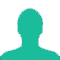# How many terms are there in 20, 25, 30......... 140

A. 22

B. 25

C. 23

D. 24

### Solution(By Examveda Team)

\eqalign{ & {\text{Number}}\,{\text{of}}\,{\text{terms}}, \cr & = {\frac{{ {{1^{st}}\,t{\text{erm - last}}\,{\text{term}}} }}{{{\text{common}}\,{\text{difference}}}}} + 1 \cr & = {140 - \frac{{20}}{5}} + 1 \cr & = {\frac{{120}}{5}} + 1 \cr & = 24 + 1 \cr & = 25 \cr}

1.Some mistake in calculation
L = a + (n-1)d where L = last term = 140
a = 20 , d= 5 , n = ?
n -1 = (L - a)/d
n = 25

2.25....

Related Questions on Progressions